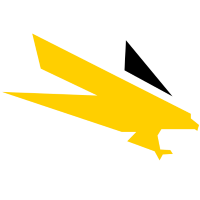# Agnico Eagle Mines Ltd (NYSE:AEM)

49.22 USD +1.02 USD ( +2.12% )Agnico Eagle Mines Ltd
NYSE:AEM

## DCF Value

Estimated DCF Value of oneAEM stock under the base case scenario is 30.9 USD. Compared to the current market price of 49.22 USD, the stock is Overvalued by 37%.

Estimated DCF Value of one NYSE:AEM stock is 30.9 USD. Compared to the current market price of 49.22 USD, the stock is Overvalued by 37% .

DCF valuation is one of two methods of placing a monetary value on a company; the other is Relative Valuation method. We use a combination of these two methods to calculate the Intrinsic Value of stock as accurately as possible.

Discount Rate
8.61%
Terminal Growth
0%
Growth Period
5 Years
Discount Rate
8.61%
Terminal Growth
0%
Growth Period
5 Years

You can change any inputs, such as future revenue, using Present Value Calculation block.

DCF Valuation FAQ:
AEM DCF Value
Base Case
30.9 USD
Overvaluation 37%
DCF Value
PriceAgnico Eagle Mines Ltd Competitors:
DCF Valuation
AU
AngloGold Ashanti Ltd
LECBF
Lepanto Consolidated Mining Co
NEM
Newmont Corporation

## DCF Value Calculation

### Capital Structure From Present Value to DCF ValuePresent Value 14B USD + Cash & Equivalents 1.1B USD + Investments 453M USD Firm Value 15.5B USD - Debt 1.5B USD Equity Value 14.1B USD / Shares Outstanding 456M AEM DCF Value 30.9 USD
Overvalued by 37%

To view the process of calculating the Present Value of Agnico Eagle Mines Ltd' future free cash flow, see the Present Value Calculation block.

## Present Value Calculation

### Discounted Cash Flow Model Present Value of Free Cash FlowRotate your device for better experience.
Currency: USD

To view the process of deriving the DCF Value of one share from the estimated Present Value, see the DCF Value Calculation block.

## Sensitivity Analysis

### Sensitivity Analysis DCF Value Sensitivity AnalysisAnalyze the possible values of AEM stock DCF Value given various model inputs such as Revenue Growth, Operating Margin, and Discount Rate.

Similar Stocks

## AEM Stock DCF Valuation FAQ

What is the DCF value of one AEM stock?

Estimated DCF Value of oneAEM stock under the base case scenario is 30.9 USD. Compared to the current market price of 49.22 USD, the stock is Overvalued by 37%.

The true DCF Value lies somewhere between the worst-case and best-case scenario values. This is because the future is not predetermined, and the stock's DCF Value is based almost entirely on the future of the company. Knowing the full range of possible stock intrinsic values gives a complete picture of the investment risks and opportunities.

How was the DCF Value calculated?

1. Present Value Calculation. Using the DCF Operating Model we projectAgnico Eagle Mines Ltd's future free cash flow and discount it at a selected discount rate to calculate its Present Value (14B USD).

2. DCF Value Calculation. We use the company's capital structure to calculate the total Equity Value based on the previously computed Present Value of the free cash flow. Dividing the Equity Value by the number of shares outstanding gives us the DCF Value of 30.9 USD per oneAEM share.